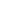GEO-1009 Three-dimensional Shapes is a Course

GEO-1009 Three-dimensional Shapes

Self-paced

Full course description

Course Description

The objects that you interact with each day are three dimensional – they have length, width, and height. In this course, you will learn about three-dimensional shapes and their properties.

By the end of this course, you will be able to

• Define three-dimensional shapes

• Calculate the surface area and volume of a prism

• Calculate the surface area and volume of a cylinder

• Calculate the surface area and volume of a regular pyramid

• Calculate the surface area and volume of a right circular cone

• Calculate the surface area and volume of a sphere

Estimated completion time (hours): 1.1